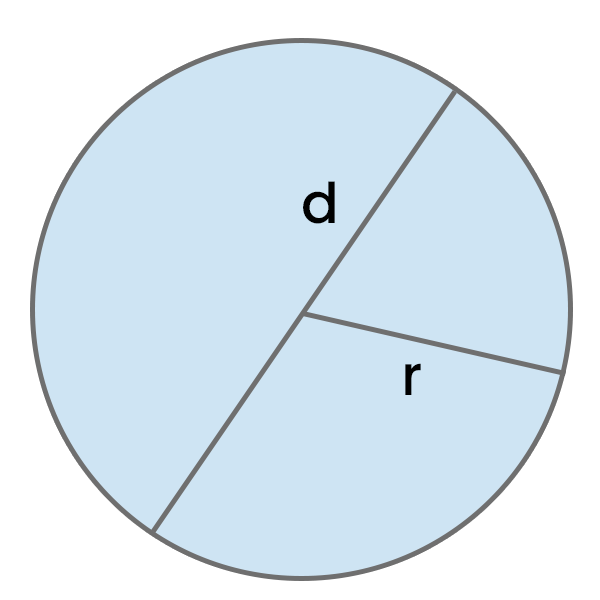# Diameter of a Circle Calculator

Calculate the diameter of a circle based on the circle radius, area of a circle, or circumference of a circle in chosen units.Result:

## How to use this calculator?

1. Select whether you want to calculate the diameter of a circle based on its radius, area, or circumference.
2. Enter the radius, area, or circumference, depending on what you selected in the field above.
3. Get your Diameter of a Circle.

## How to Calculate the Diameter of a Circle?

There are several methods to calculate the Diameter of a Circle. Choose the one that allows you to perform calculations based on the data you have.

Multiply the Circle Radius by 2.

Diameter of a Circle = 2 * Circle Radius

### Based on Circumference of a Circle

Divide Circumference of a Circle by Pi (approximately 3.14).

Diameter of a Circle = Circumference of a Circle / π

### Based on Area of a Circle

Multiply 2 by the square root of the Area of a Circle divided by Pi (approximately 3.14).

Diameter of a Circle = 2 * √(Area of a Circle / π)